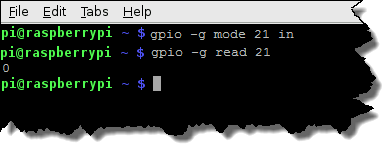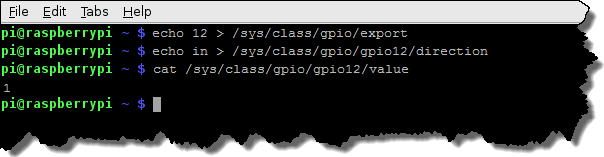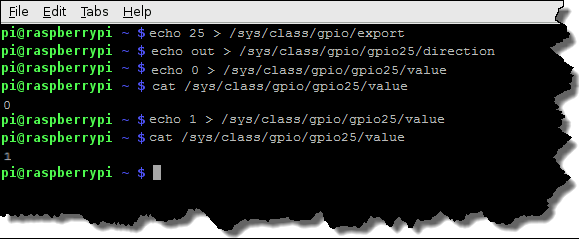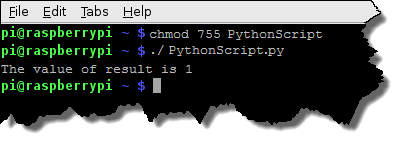For a mapping diagram and overlay for your Pi, see Pin-to-Module Mapping.

Each of these code samples uses a different method to read and write to I/O points.

You can use any Pi operating system and programming language to read and write to I/O points.

Just remember:

• Opto 22 I/O modules use negative true logic (a zero bit means On and a 1 bit means Off).
When you read and write to I/O points, 0 is On and 1 is Off.
• Before reading or writing to an I/O point, you must configure its associated GPIO pin as an input or output. (Use the Opto 22 Pin-to-Module diagram to see how GPIO pins are mapped to I/O points.)
• If the GPIO pin is mapped to an input module, make sure to configure it as input;
if it’s mapped to an output module, make sure to configure it as output.
• Once you configure the GPIO pin, you don’t need to change it (unless you swap out the Pi or connect the same carrier board to a rack with different I/O).
• You can both read and write to output points (0 for On; 1 for Off).
• For input points, you perform reads (0 is On; 1 is Off).
If you accidentally write to an input point, no problem—nothing will happen.

Top

## Terminal

You can issue read and write commands from a terminal.

The examples in this section show LXTerminal on a Pi running Raspbian.

A value of 0 means the I/O point is On; 1 means it’s Off.

Syntax

``````gpio readall
``````

In this image, highlighted GPIO pins are mapped to I/O points.Don’t let the column names in this chart confuse you. In a Readall chart from LXTerminal, the GPIO pin numbers are in the column labeled “BCM.”

For a mapping diagram and overlay for your Pi, see Pin-to-Module Mapping.This example:

• Configures GPIO pin 21 as input.
• Reads the input point mapped to GPIO 21 and writes its value to the terminal.
The input point is at rack position 0, and its status is 0 (On).

Syntax

``````gpio -g mode [GPIO pin number] in
gpio -g read [GPIO pin number]
``````

### Write to outputThis example:

• Configures GPIO pin 20 as output.
• Turns On the output point mapped to the GPIO.
• Reads and writes the point’s value to the terminal.
• Turns Off the point.
• Reads and writes the point’s value.

Syntax

``````gpio -g mode [GPIO pin number] out
gpio -g write [GPIO pin number] 0
gpio -g read [GPIO pin number]
gpio -g write [GPIO pin number] 1
gpio -g read [GPIO pin number]
``````

Top

## The Pi Filesystem

The Pi filesystem is accessible from any programming language and also from a terminal.

The files that control GPIO pins are located in `/sys/class/gpio`.This example:

• Configures the GPIO as input.
• Reads the input point mapped to GPIO 12 and writes its value to the terminal.
(The input point is at rack position 7 and its status is 1 (Off).)

Syntax

``````echo [GPIO pin number] > /sys/class/gpio/export
echo in > /sys/class/gpio/gpio[GPIO pin number]/direction
cat /sys/class/gpio/gpio[GPIO pin number]/value
``````

### Write to outputThis example:

• Configures the GPIO as output.
• Turns On the output point mapped to the GPIO.
• Reads and writes the point’s value to the terminal.
• Turns Off the point.
• Reads and writes the point’s value.

Syntax

``````echo [pin number] > /sys/class/gpio/export
echo out > /sys/class/gpio/gpio[pin number]/direction
echo 0 > /sys/class/gpio/gpio[pin number]/value
cat /sys/class/gpio/gpio25/value
echo 1 > /sys/class/gpio/gpio[pin number]/value
cat /sys/class/gpio/gpio25/value
``````

Top

## RPi.GPIO Python Library

If you code in Python, the RPi.GPIO Python library (included with Raspbian) lets you configure, read, and write to GPIO pins.

We’ve created a Python script that shows how to read and write with the RPi.GPIO library.

• To download the script to the current directory using a terminal, enter `wget http://developer.opto22.com/downloads/PythonScript.py`

The comments in the script point out where it:

• Invokes the Python interpreter
• Includes the RPi.GPIO library and sets GPIO as a local name in the script
• Sets the pin-numbering scheme for the script
(The `GPIO.BOARD` option means that that you’re referring to the pins by their physical pin number. To refer to the pins by their GPIO pin number, use the `GPIO.BCM` option.)
• Configures physical pin number 13 as input.
Note: Physical pin 13 is mapped to rack position 10.
• Reads the input point mapped to pin 13 and stores it in a variable.
• Prints the point’s value to the terminal.
• Configures physical pin number 15 as output.
Note: Physical pin 15 is mapped to rack position 11.
• Turns On the output point mapped to pin 15.
• Turns Off the output point mapped to pin 15.
The script assumes you have a 16-module rack, an input module in rack position 10, and an output module in position 11. The script uses physical pin numbers and not GPIO pin numbers or I/O module rack positions, but you can edit the script to use GPIO pin numbers.

To run the script:

1. If necessary, modify the pin numbers in the script to correspond to I/O modules on your rack.
2. Make the script executable by entering ` chmod 755 PythonScript.py`
3. Run the script by entering ` ./PythonScript.py `

The output looks something like this:Top

To simplify accessing I/O when you write your own code, we’ve written a Python script that your code can reference.

• To download the script to the current directory using a terminal, enter `wget http://developer.opto22.com/downloads/Opto22.PI.py`

The script references an I/O module’s rack position to read and write to its point, and to configure its associated GPIO pin. It also contains comments to help you understand what it does.

Examples

### How to run Opto22.PI.py

Open a terminal, and then run the script using this syntax:

`sudo python Opto22.PI.py [module number] [command] [value]`

• [module number] : Rack position of the target I/O module (0 through 15).
• [command] :
• config configures the I/O module's associated GPIO pin as either input or output.
• write turns an output point On or Off.
• [value] :
• If [command] is blink, for [value], type the number of seconds to blink. The minimum value is 2 (blink on for 1 second; blink off for 1 second).

• If [command] is config, [value] is `input` or `output`.
You must configure the GPIO pin to match the I/O module's type.
• If the GPIO pin is mapped to an input module, enter `input` for the value.
• If the pin is mapped to an output module, enter `output` for the value.
• If [command] is read, you don't need the [value] argument. Leave it blank.

• If [command] is write, [value] is `1` for On or `0` for Off.

### Examples for LXTerminal

Configure the GPIO pin mapped to rack position 0 as input

``````sudo python Opto22.PI.py 0 config input
``````

Read the I/O module at rack position 3

``````sudo python Opto22.PI.py 3 read
``````

Turn Off the I/O module at rack position 15
(Remember that in Opto22.PI.py, 1 = On and 0 = Off)

``````sudo python Opto22.PI.py 15 write 0
``````

Top